Courses

# Test: Factorisation- 1

## 20 Questions MCQ Test Mathematics (Maths) Class 8 | Test: Factorisation- 1

Description
This mock test of Test: Factorisation- 1 for Class 8 helps you for every Class 8 entrance exam. This contains 20 Multiple Choice Questions for Class 8 Test: Factorisation- 1 (mcq) to study with solutions a complete question bank. The solved questions answers in this Test: Factorisation- 1 quiz give you a good mix of easy questions and tough questions. Class 8 students definitely take this Test: Factorisation- 1 exercise for a better result in the exam. You can find other Test: Factorisation- 1 extra questions, long questions & short questions for Class 8 on EduRev as well by searching above.
QUESTION: 1

### Divide as directed: 5 (2x + 1) (3x + 5) ÷ (2x + 1)

Solution: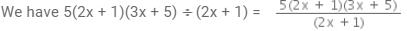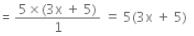∴  5(2x + 1)(3x + 5)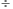(2x + 1) = 5(3x+5)

QUESTION: 2

### Divide as directed: 26xy (x + 5) (y – 4) ÷ 13x (y – 4)

Solution:

We have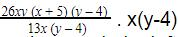gets cancelled,and we have 13*2=26, so the remainder left is 2y(x+5).

QUESTION: 3

### Find and correct the errors in the following mathematical statements. 4 (x – 5) = 4x – 5

Solution:

By the distributive property a(b-c)=ab- ac
so 4(x-5)=4x-20

QUESTION: 4

Find and correct the errors in the following mathematical statements.

x (3x + 2) = 3x2 + 2

Solution:

x (3x + 2)= 3x2+2x (by distributive property a(b+c)=ab+ac)

QUESTION: 5

Factorise: 10x− 18x+ 14x4

Solution:

10x2 − 18x3 + 14x= 2x(5x− 9x2 + 7x3) = 2x2(5− 9x + 7x2)

QUESTION: 6

Factorise: x2+xy+ 8x+ 8y

Solution:

x^2 + xy + 8x + 8y = x[x + y] + 8[x + y]

= (x + y)(x + 8)

QUESTION: 7

Factorise: 4y−12y + 9

Solution:

We have 4y- 12y+9. comparing the equation with (a-b)2=a2-2ab+b2,gives us a2=(2y)2,2ab=2*3*2y and b2=(3)2.Hence the answer is (2y-3)2.

QUESTION: 8

Solve: 7x2y2z÷ 14xyz

Solution:

7x²y²z²÷14xyz
=(7/14)(x²/x)(y²/y)(z²/z)
=1/2(x)(y)(z)
=1/2xyz.

QUESTION: 9

Find the common factors of 2y, 22xy.

Solution:

Only 2y is common in both the terms

QUESTION: 10

The _______ may be numbers, algebraic variables or algebraic expressions.

Solution:

Factors are numbers we can multiply together to get another number. A constituent or element that brings about certain effects or results, or indicates a specific multiple, number, or quantity.

QUESTION: 11

Factorise : x4 - (y+z)4

Solution:

x4- (y+z)4, applying x- y2=(x-a)(x+a), gives (x2-(y+z)2)(x2+(y+z)2). Again applying the same identity gives (x-(y+z))(x+(y+z))(x2+(y+z)2)

QUESTION: 12

Factorise: x4− (x−z)4

Solution:

x4-(x-z)= (x2-(x-z)2)(x2+(x-z)2) = (x-(x-z))(x+(x-z)(x2+(x-z)2) = z(2x-z)(x2+(x-z)2(Applying a- b= (a+b)(a-b))

QUESTION: 13

Divide the given polynomial by the given monominal: 8 (x3y2z2 + x2y3z2 + x2y2z3) ÷ 4x2y2z2

Solution:

8 (x3y2z2 + x2y3z2 + x2y2z3) ÷ 4x2y2z2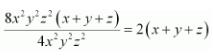QUESTION: 14

Divide the given polynomial by the given monomial: (x3+ 2x2+ 3x) ÷ 2x

Solution:

(x3+2x2+3x)/2x
=x(x2+2x+3)/2x
=(x2+2x+3)/2

QUESTION: 15

Divide as directed: 52pqr (p + q) (q + r) (r + p) ÷104pq (q + r) (r + p)

Solution: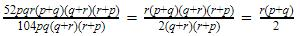QUESTION: 16

Divide as directed: 20 (y+ 4) (y2+ 5y+ 3) ÷ 5(y+ 4)

Solution: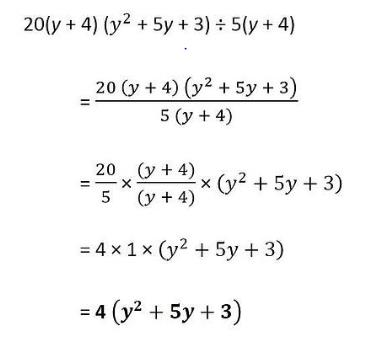QUESTION: 17

Find and correct the errors in the following mathematical statements. 2x + 3y = 5xy

Solution:

LHS = 2x + 3y
RHS = 5xy
LHS ≠ RHS
Correct statement would be
2x + 3y = 2x+3y

QUESTION: 18

Find and correct the errors in the following mathematical statements. x + 2x + 3x = 5x

Solution:

x+2x+3x=6x

QUESTION: 19

Factorise: 12x + 36

Solution:

Let us take 12 as common
and then the factor is 12(x+3) .

QUESTION: 20

Factorise: 15xy – 6x + 5y – 2

Solution:

15xy - 6x + 5y - 2 = 3x[5y-2] + 1[5y-2]
= (5y - 2)(3x + 1)